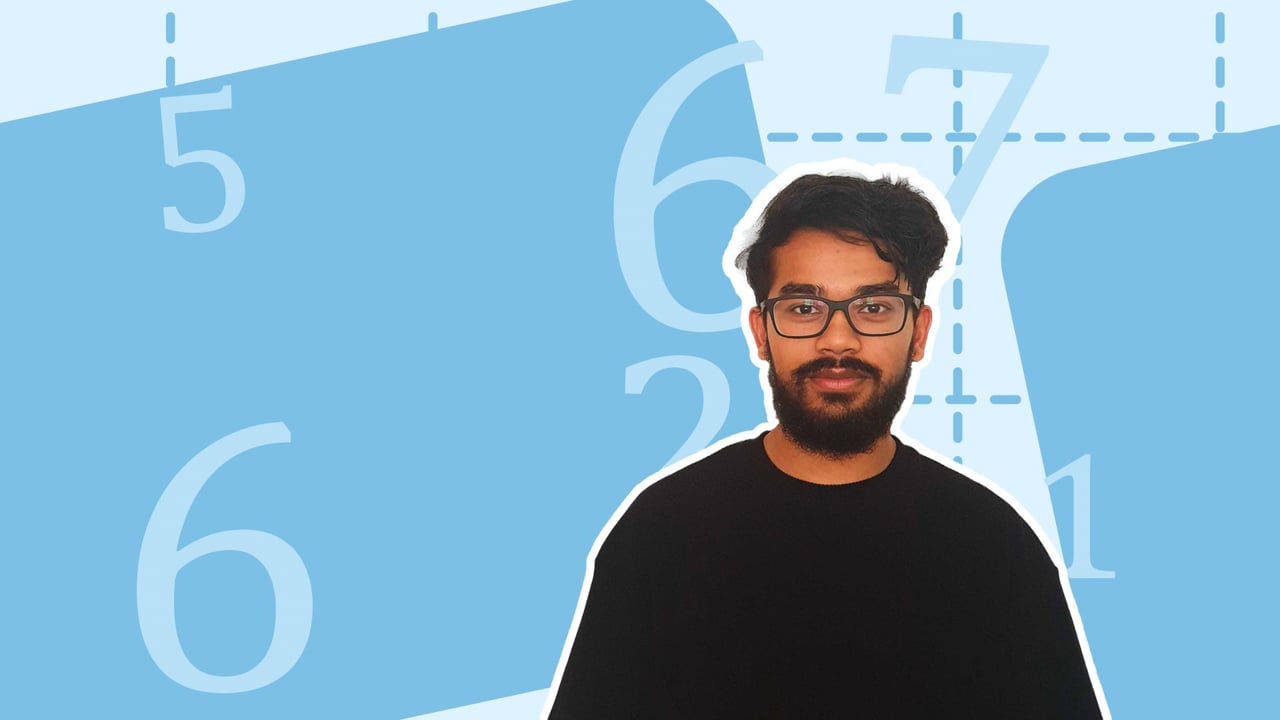Chapter OverviewMaths

Exam board

Pearson Edexcel

Number

Algebra

Graphs

Ratio proportion and rates of change

Shapes and area

Angles and geometry

Trigonometry

Probability

Statistics

Maths

# Estimation0%

Summary

# Estimation

## ​​In a nutshell

Estimation means finding an approximate answer to a calculation. Estimating involves rounding numbers to $1$ or $2$ significant figures before performing a calculation. The estimated answer would be close to the actual answer. ​

## Estimation in calculations

Round the numbers in the calculation to $1$ or $2$​ significant figures before performing the calculation to find an approximate answer.​

##### Example 1

Estimate the value of  $\dfrac{67.9+2.16}{14.4}$.

First, round all $3$ numbers to an appropriate number of significant figures:

$67.9 \mapsto 68$

$2.16 \mapsto 2$

$14.4 \mapsto 14$​​​​

Carry out the calculation with the rounded numbers:

$\dfrac{67.9+2.16}{14.4}\approx \dfrac{68+2}{14} = \dfrac{70}{14} = 5$

$\underline{\dfrac{67.9+2.16}{14.4} \approx 5}$​​

## Estimation with square roots

Square roots can be estimated by identifying square numbers near a number.

##### Example 2

Estimate the value of $\sqrt{74}$ to $1\space d.p.$

$74$ is inbetween the square numbers $64$ and $81$

$\sqrt {64} = 8 \\\sqrt {81}= 9$

$74$ is closer to $81$ than $64$.

Therefore, $\underline{\sqrt{74} \approx 8.7}$​​

​​

## Want to find out more? Check out these other lessons!

Rounding errors and estimating

FAQs

• Question: What is estimation?

• Question: How do you find the estimate for square roots?

Answer: Estimates for square roots can be found by identifying the position of numbers between consecutive square numbers.

• Question: How do you estimate calculations?

Answer: Round the numbers to an appropriate number of significant figures, then calculate with these numbers.

Theory

Exercises